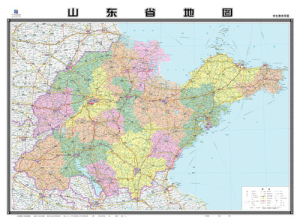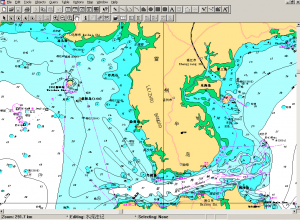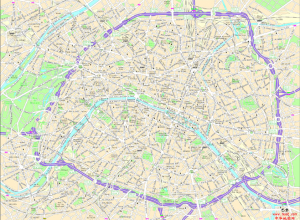电子版 +  0% 印刷 +  15%+印费 出版 +  30%
 全国图集 +  30% 专题图集 +  10% 世界/国外 +  20%电子版 +  0% 印刷 +  15%+印费 出版 +  30%
 挂图 +  30% +外文 +  10%电子版 +  0% 印刷 +  15%+印费 出版 +  30%
 挂图 +  30% +外文 +  10%电子版 +  0% 印刷 +  15%+印费 出版 +  30%
 全国图 +  20% 世界/国外 + 30%电子版 +  0% 挂图 + 20% 印刷 +  15%+印费 出版 +  30%
 全国图 +  30% 世界/国外 + 40%电子版 +  0% 印刷 +  15%+印费 出版 +  30%
 全国图 +  30% 世界/国外 + 40%电子版 +  0% 印刷 +  15%+印费电子版 +  0% 印刷 +  15%+印费电子版 +  0% 印刷 +  15%+印费 出版 +  30%电子版 +  0% 印刷 +  15%+印费 出版 +  30%